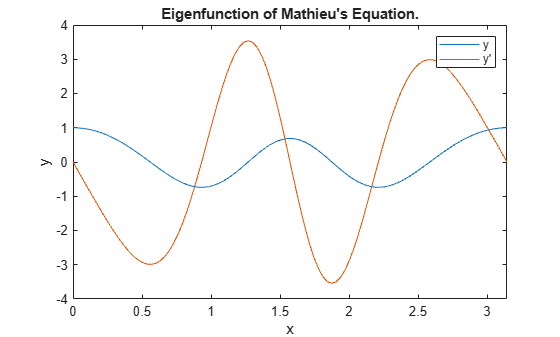# Solve BVP with Unknown Parameter

This example shows how to use `bvp4c` to solve a boundary value problem with an unknown parameter.

Mathieu's equation is defined on the interval $\left[0,\pi \right]$ by

${{\mathit{y}}^{\prime }}^{\prime }+\left(\lambda -2\mathit{q}\text{\hspace{0.17em}}\mathrm{cos}\left(2\mathit{x}\right)\right)\mathit{y}=0$.

When the parameter $\mathit{q}=5$, the boundary conditions are

${\mathit{y}}^{\prime }\left(0\right)=0$,

${\mathit{y}}^{\prime }\left(\pi \right)=0$.

However, this only determines $\mathit{y}\left(\mathit{x}\right)$ up to a constant multiple, so a third condition is required to specify a particular solution,

$\mathit{y}\left(0\right)=1$.

To solve this system of equations in MATLAB, you need to code the equations, boundary conditions, and initial guess before calling the boundary value problem solver `bvp4c`. You can either include the required functions as local functions at the end of a file (as done here), or save them as separate, named files in a directory on the MATLAB path.

### Code Equation

Create a function to code the equations. This function should have the signature `dydx = mat4ode(x,y,lambda)`, where:

• `x` is the independent variable.

• `y` is the dependent variable.

• `lambda` is an unknown parameter representing an eigenvalue.

You can write Mathieu's equation as a first-order system using the substitutions ${\mathit{y}}_{1}=\mathit{y}$ and ${\mathit{y}}_{2}={\mathit{y}}^{\prime }$,

${{\mathit{y}}_{1}}^{\prime }={\mathit{y}}_{2}$,

${{\mathit{y}}_{2}}^{\prime }=-\left(\lambda -2\mathit{q}\text{\hspace{0.17em}}\mathrm{cos}\left(2\mathit{x}\right)\right){\mathit{y}}_{1}$.

The corresponding function is then

```function dydx = mat4ode(x,y,lambda) % equation being solved dydx = [y(2) -(lambda - 2*q*cos(2*x))*y(1)]; end ```

Note: All functions are included as local functions at the end of the example.

### Code Boundary Conditions

Now, write a function that returns the residual value of the boundary conditions at the boundary points. This function should have the signature `res = mat4bc(ya,yb,lambda)`, where:

• `ya` is the value of the boundary condition at the beginning of the interval $\left[\mathit{a},\mathit{b}\right]$.

• `yb` is the value of the boundary condition at the end of the interval $\left[\mathit{a},\mathit{b}\right]$.

• `lambda` is an unknown parameter representing an eigenvalue.

This problem has three boundary conditions in the interval $\left[0,\pi \right]$. To calculate the residual values, you need to put the boundary conditions into the form $\mathit{g}\left(\mathit{x},\mathit{y}\right)=0$. In this form the boundary conditions are

${\mathit{y}}^{\prime }\left(0\right)=0$,

${\mathit{y}}^{\prime }\left(\pi \right)=0$,

$\mathit{y}\left(0\right)-1=0$.

The corresponding function is then

```function res = mat4bc(ya,yb,lambda) % boundary conditions res = [ya(2) yb(2) ya(1)-1]; end ```

### Create Initial Guess

Lastly, create an initial guess of the solution. You must provide an initial guess for both solution components ${\mathit{y}}_{1}=\mathit{y}\left(\mathit{x}\right)$ and ${\mathit{y}}_{2}={\mathit{y}}^{\prime }\left(\mathit{x}\right)$, as well as the unknown parameter $\lambda$.

For this problem, a cosine function makes for a good initial guess since it satisfies the three boundary conditions. Code the initial guess for $\mathit{y}$ using a function that returns the guess for ${\mathit{y}}_{1}$ and ${\mathit{y}}_{2}$.

```function yinit = mat4init(x) % initial guess function yinit = [cos(4*x) -4*sin(4*x)]; end ```

Call `bvpinit` using a mesh of 10 points in the interval $\left[0,\pi \right]$, the initial guess function, and a guess of 15 for the value of $\lambda$.

```lambda = 15; solinit = bvpinit(linspace(0,pi,10),@mat4init,lambda);```

### Solve Equation

Call `bvp4c` with the ODE function, boundary condition function, and initial guess.

`sol = bvp4c(@mat4ode, @mat4bc, solinit);`

### Value of Parameter

Print the value of the unknown parameter $\lambda$ found by `bvp4c`. This value is the fourth eigenvalue ($\mathit{q}=5$) of Mathieu's equation.

```fprintf('Fourth eigenvalue is approximately %7.3f.\n',... sol.parameters)```
```Fourth eigenvalue is approximately 17.097. ```

### Plot Solution

Use `deval` to evaluate the solution computed by bvp4c at 100 points in the interval $\left[0,\pi \right]$.

```xint = linspace(0,pi); Sxint = deval(sol,xint);```

Plot both solution components. The plot shows the eigenfunction (and its derivative) associated with the fourth eigenvalue ${\lambda }_{4}=17.097$.

```plot(xint,Sxint) axis([0 pi -4 4]) title('Eigenfunction of Mathieu''s Equation.') xlabel('x') ylabel('y') legend('y','y''')```### Local Functions

Listed here are the local helper functions that the BVP solver `bvp4c` calls to calculate the solution. Alternatively, you can save these functions as their own files in a directory on the MATLAB path.

```function dydx = mat4ode(x,y,lambda) % equation being solved q = 5; dydx = [y(2) -(lambda - 2*q*cos(2*x))*y(1)]; end %------------------------------------------- function res = mat4bc(ya,yb,lambda) % boundary conditions res = [ya(2) yb(2) ya(1)-1]; end %------------------------------------------- function yinit = mat4init(x) % initial guess function yinit = [cos(4*x) -4*sin(4*x)]; end %-------------------------------------------```